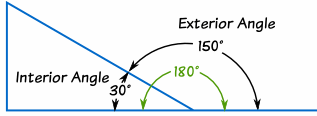# what is exterior angle property?

Dear Student!

The angle formed by a side with its extended adjacent side of a triangle is called an exterior angle of the triangle.
Exterior angle property states that the measure of exterior angles of a triangle is equal to the sum of its two interior opposite angles.
Example:
Find the value of x in the given figure.Solution:
Exterior angle = Sum of opposite interior angles
x = 60° + 60°
x = 120°

Cheers!

• 15An Exterior Angle

An angle which is present outside a shape is known as exterior angle.

(Dont forget to vote)

• 8

An exterior angle of a triangle is equal to the sum of its interior opposite angles.

• 3

The exterior angle theorem is a theorem in elementary geometry which states that the exterior angle of a triangle is equal to the sum of the two remote interior angles.

A triangle has three corners, called vertices. The sides of a triangle that come together at a vertex form an angle. This angle is called the interior angle. In the picture below, the angles a, b and c are the three interior angles of the triangle. An exterior angle is formed by extending one of the sides of the triangle; the angle between the extended side and the other side is the exterior angle. In the picture, angle d is an exterior angle.

The exterior angle theorem says that the size of an exterior angle at a vertex of a triangle equals the sum of the sizes of the interior angles at the other two vertices of the triangle. So, in the picture, the size of angle d equals the size of angle a plus the size of angle c.Given: In ∆ABC, angle ACD is the exterior angle.

Proof:

StatementsReason
In ∆ABC,a +b +c = 180°------Sum of the measures of all the angles of a triangle is 180°
Also,b +d = 180°-------Linear pair axioma +c +b =b +dFrom  anda +c +b =b +mdd =a +c
i.e.ACD =ABC +BAC

Hence, proved.

Best Wishes !@

• 2

exterior angle is th esum of the opposite angles

• -3